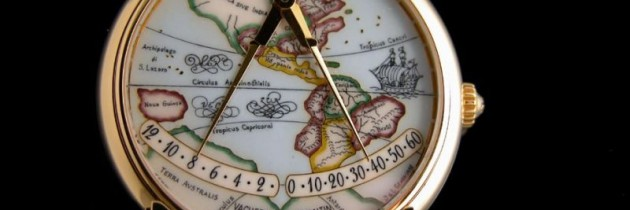Posted by on 6th July, 2009# Vacheron Constantin watch experiment..

This morning I ran across an article about some watches and there is one that caught my attention. It is a Vacheron Constantin Mercator America and it is a beautiful watch. A bit pricey, but if I ever have \$47.500 that I don’t need, maybe I’ll get it some day.

The hands of the clock represent what are called nautical dividers and are used by navigational officers to quickly determine distance and range on a maritime charts. I have used these plenty, so perhaps that explains the fascination with this watch.

You can buy the watch here and find some exquisite information here. In the meantime, I could only think that the display was quite interesting, and having a maritime background, I loved the concept and originality of its design.

I have added a “seconds” counter that moves along the bottom edge of the numbers as well. This was a relatively easy experiment as one can tell by the code…

### armhour.xaml.cs

```using System;
using System.Collections.Generic;
using System.Linq;
using System.Net;
using System.Windows;
using System.Windows.Controls;
using System.Windows.Documents;
using System.Windows.Input;
using System.Windows.Media;
using System.Windows.Media.Animation;
using System.Windows.Shapes;
namespace silverlight_clock_vacheron
{
public partial class ArmHour : UserControl
{
private RotateTransform rotateTransform = new RotateTransform();
public ArmHour()
{
InitializeComponent();
//Moves the canvas down and left
TranslateTransform translateTransform = new TranslateTransform();
translateTransform.X = 190;
translateTransform.Y = 89;
this.RenderTransform = translateTransform;
}
internal double setAngle(DateTime _now){
int _hour = _now.Hour;
int _minute = _now.Minute;
if (_hour == 12)
{
_hour = 0;
}
else if (_hour > 12)
{
_hour -= 12;
}
//calculate fraction
double hourFull = (double)_hour * (45.00 / 12.00);
double hourFraction = (double)_minute * (3.75 / 60);
this.rotateTransform.Angle = hourFull + hourFraction;
this.ImageArmHour.RenderTransform = rotateTransform;
return this.rotateTransform.Angle;
}
}
}
```

### armminute.xaml.cs

```using System;
using System.Collections.Generic;
using System.Linq;
using System.Net;
using System.Windows;
using System.Windows.Controls;
using System.Windows.Documents;
using System.Windows.Input;
using System.Windows.Media;
using System.Windows.Media.Animation;
using System.Windows.Shapes;
namespace silverlight_clock_vacheron
{
public partial class ArmMinute : UserControl
{
private RotateTransform rotateTransform = new RotateTransform();
public ArmMinute()
{
InitializeComponent();
//Moves the canvas down and left
TranslateTransform translateTransform = new TranslateTransform();
translateTransform.X = 190;
translateTransform.Y = 89;
this.RenderTransform = translateTransform;
}
internal double setAngle(DateTime _now)
{
int _minute   = _now.Minute;
int _seconds  = _now.Second;
//calculate fraction
double minuteFull   = (double)  _minute * (-45.00 / 60.00);
double minuteFraction= (double)_seconds * ((3.75/5.00) / 60.00);
this.rotateTransform.Angle = minuteFull-minuteFraction;
this.ImageArmMinute.RenderTransform = rotateTransform;
return this.rotateTransform.Angle;
}
}
}
```

### armsecond.xaml.cs

```using System;
using System.Collections.Generic;
using System.Linq;
using System.Net;
using System.Windows;
using System.Windows.Controls;
using System.Windows.Documents;
using System.Windows.Input;
using System.Windows.Media;
using System.Windows.Media.Animation;
using System.Windows.Shapes;
namespace silverlight_clock_vacheron
{
public partial class ArmSecond : UserControl
{
private RotateTransform rotateTransform = new RotateTransform();
public ArmSecond()
{
InitializeComponent();
//Moves the canvas down and left
TranslateTransform translateTransform = new TranslateTransform();
translateTransform.X = 190;
translateTransform.Y = 89;
this.RenderTransform = translateTransform;
}
internal double setAngle(DateTime _now)
{
int _seconds = _now.Second;
int _milliseconds = _now.Millisecond;
//calculate fraction
double secondFull   = (double)_seconds*(-45.00/60.00);
double secondFraction= ((double)_milliseconds/1000.00)*(3.75/5.00);
this.rotateTransform.Angle = secondFull - secondFraction;
this.ImageArmSecond.RenderTransform = rotateTransform;
return this.rotateTransform.Angle;
}
}
}
```